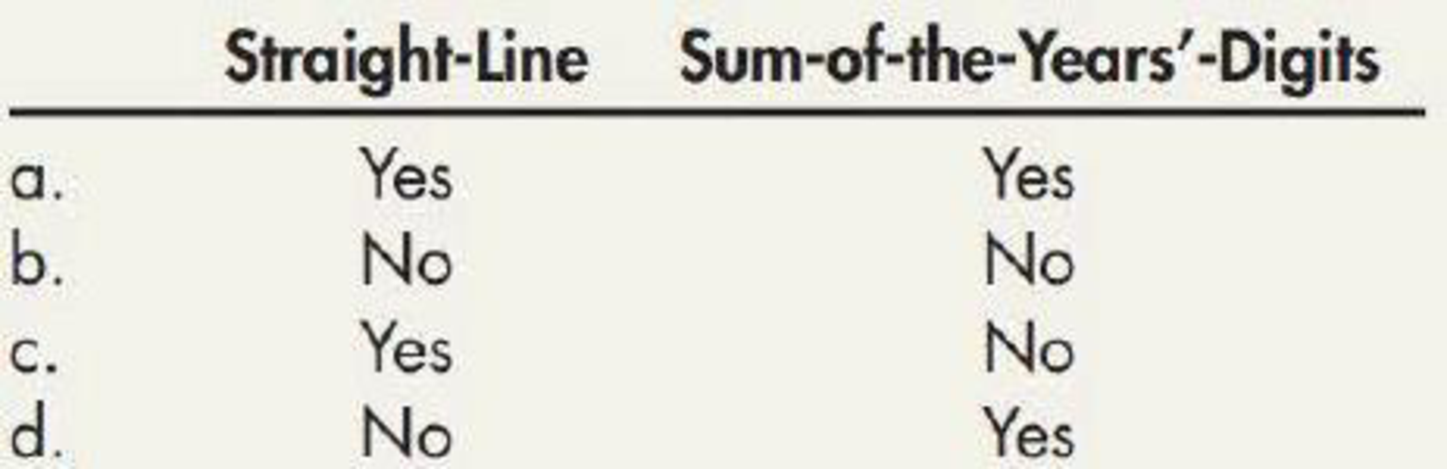Chapter 11, Problem 6MC### Intermediate Accounting: Reporting...

3rd Edition
James M. Wahlen + 2 others
ISBN: 9781337788281

#### Solutions

Chapter
Section### Intermediate Accounting: Reporting...

3rd Edition
James M. Wahlen + 2 others
ISBN: 9781337788281
Textbook Problem
3 views

# At the end of the expected useful life of a depreciable asset with an estimated 15% residual value, the accumulated depreciation would equal the original cost of the asset under which of the following depreciation methods?To determine

Identify the correct option for the given statement.

Explanation

Straight-line depreciation method: The depreciation method which assumes that the consumption of economic benefits of long-term asset could be distributed equally throughout the useful life of the asset is referred to as straight-line method.

Sum-of- the-years’ digits method:

Sum-of-the years’ digits method determines the depreciation expense by multiplying the depreciable base and declining fraction.

In this case, the accumulated depreciation would not equal the original cost of the asset under any situation...

### Still sussing out bartleby?

Check out a sample textbook solution.

See a sample solution

#### The Solution to Your Study Problems

Bartleby provides explanations to thousands of textbook problems written by our experts, many with advanced degrees!

Get Started

#### Fill in the blanks:

Principles of Economics (MindTap Course List)

#### Does interest rate parity imply that interest rates are the same in all countries?

Fundamentals of Financial Management, Concise Edition (MindTap Course List)

#### Refer to an online finance source such as Yahoo! Finance or Google Finance to look up the P/E ratios for Verizo...

Fundamentals of Financial Management, Concise Edition (with Thomson ONE - Business School Edition, 1 term (6 months) Printed Access Card) (MindTap Course List)